#### Plenary speakers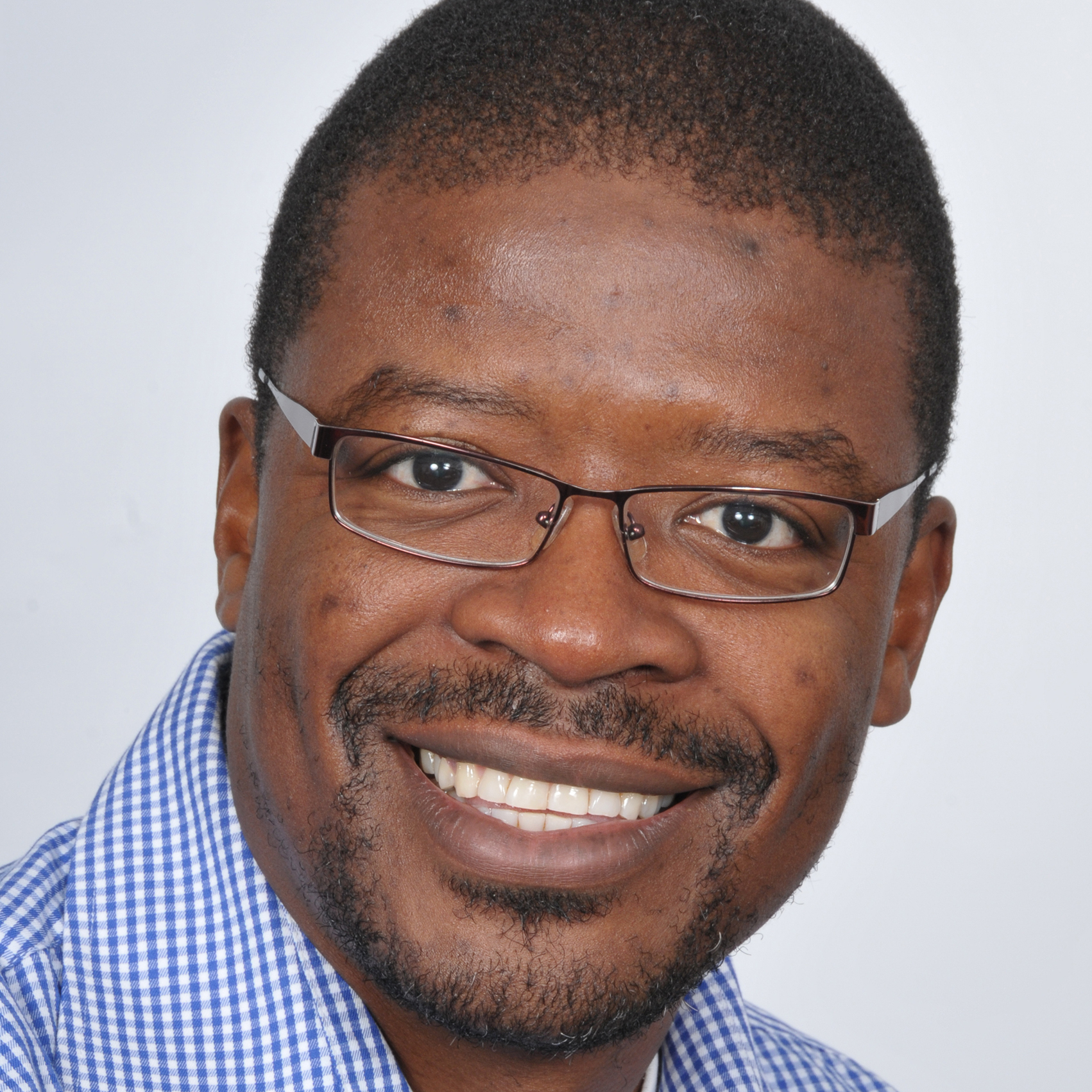##### Mapundi Banda (University of Pretoria)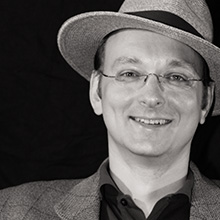##### Folkmar Bornemann (TU Munich)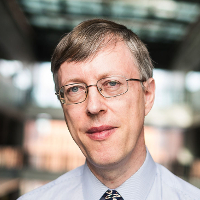##### Nick Higham (University of Manchester)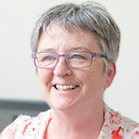##### Elizabeth Mansfield (University of Kent)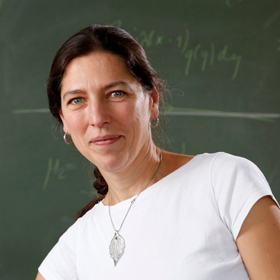##### Beatrice Pelloni(University of Reading)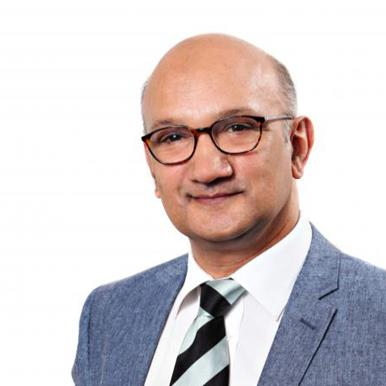##### Daya Reddy(University of Cape Town)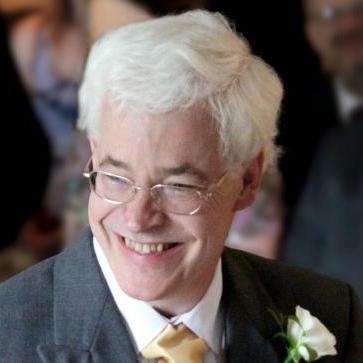##### Nick Trefethen(University of Oxford)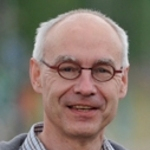##### Walter Van Assche(KU Leuven)
• Mapundi Banda (University of Pretoria)
• Optimal control and inverse problems for evolution models
Optimal control and inverse problems governed by partial differential equations will be considered. The first part of the presentation will derive continuous and discrete schemes for optimal control problems subject to nonlinear, hyperbolic conservation laws. The initial condition will be treated as the control and a tracking-type problem also with non-smooth desired states will be approximated. The second part will consider a simultaneous identification of a coefficient and an initial condition of a partial differential equation from boundary measurement problem. An identification algorithm will be discussed. Some numerical simulations to show the effectiveness of the proposed algorithm will also be demonstrated.
• Folkmar Bornemann (TU Munich)
• Numerical Problems Inspired by Discrete Complex Analysis
Discrete Complex Analysis, that is, the quest for discretizing the whole theory and not just single manifestations of it has led to a wealth of interesting mathematical concepts and a rich nonlinear theory related to integrable systems. A major question is the construction of discrete analogues of the set of "standard" holomorphic maps and a good model problem is the power map z^a. The stable numerical evaluation of that map touches on many mathematical topics ranging from boundary value problems of discrete Painlevé equations to infinite-dimensional linear algebra and discrete optimization.
• Nick Higham (University of Manchester)
• Challenges in Multivalued Matrix Functions

Multivalued matrix functions arise in solving various kinds of matrix equations. The matrix logarithm is the prototypical example. Another example is the Lambert W function of a matrix, which is much less well known but has been attracting recent interest. A theme of the talk is the importance of choosing appropriate principal values and making sure that the correct choices of signs and branches are used, both in theory and in computation. We will give examples where incorrect results have previously been obtained.

We focus on matrix inverse trigonometric and inverse hyperbolic functions, beginning by investigating existence and characterization. Turning to the principal values, various functional identities are derived, some of which are new even in the scalar case, including a "round trip'' formula that relates acos(cos A) to A and similar formulas for the other inverse functions. Key tools used in the derivations are the matrix unwinding function and the matrix sign function.

A new inverse scaling and squaring type algorithm employing a Schur decomposition and variable-degree Padé approximation is derived for computing acos, and it is shown how it can also be used to compute asin, acosh, and asinh. In numerical experiments the algorithm is found to behave in a forward stable fashion and to be superior to computing these functions via logarithmic formulas.

• Elizabeth Mansfield (University of Kent)
• Lattice based Multispace and Applications

In this talk I will describe the construction of a manifold called "multispace" which uses the Lagrange interpolation data of functions as coordinates and which has the jet space for functions embedded as a sub manifold.

The purpose for the construction is the simultaneous calculation of smooth and discrete invariants of Lie group actions on the base space, via a moving frame, together with their differential (smooth) and recurrence (discrete) relations, with all appropriate continuum limits guaranteed by the construction.

Two main applications have been described to date, to discrete integrable systems and to discrete variational systems. I will discuss the application to variational shallow water systems, showing how a simultaneous smooth and discrete

Noether's theorem leads to the conservation of energy and momenta in the discrete scheme, with guaranteed continuum limits to the smooth energy and momenta.

This is joint work with Gloria Mari Beffa (U Wisconsin-Madison).

• Beatrice Pelloni (University of Reading)
• Beyond integrability: the far-reaching consequences of thinking about boundary conditions

In this talk, I will outline results obtained in the last fifteen years, all stemming from the original aim to include boundary conditions into the celebrated Inverse Scattering Transform, which is in essence a nonlinear Fourier transform.

The talk will revisit the Fourier transform on R, embedding it in a general way of thinking about integral transform that relies on a formulation in the complex domain (called a Riemann-Hilbert formulation) and start from this idea to describe a generalised approach, now known as the unified transform, or Fokas transform. This circle of ideas has produced unexpected and very general results for the solution of linear boundary value problems, including interface and moving boundary problems, new ideas for numerical schemes, a way to study of nonselfadjoint differential operators, as well as going some way to achieving the original aim of solving nonlinear integrable problems in domains with boundaries.

I will describe the key ideas and some of the most unexpected results.

• Daya Reddy (University of Cape Town)
• Discontinuous Galerkin Methods: An Overview and Some Applications

Discontinuous Galerkin (DG) methods, as the name suggests, are a class of Galerkin finite element methods in which the condition of continuity of functions across element boundaries is relaxed. DG methods have become a powerful tool in the approximate solution of hyperbolic problems, the context in which they were first developed. DG methods have also been applied with much success to elliptic PDEs and systems, and it is this class of applications that will be the focus of this presentation. A simple model problem will serve as the vehicle for motivating the DG approach and comparing it to the conventional finite element method. A further application will be in the context of problems arising in solid and fluid mechanics, and in which dependence on a parameter may lead to singular behaviour of the approximate solution. It will be shown how uniformly convergent DG approaches can be constructed in such situations.

• Nick Trefethen (University of Oxford)
• Ten things you should know about quadrature

Quadrature is the term for the numerical evaluation of integrals. It's a beautiful subject because it's so accessible, yet full of conceptual surprises and challenges. This talk will review ten of these, with plenty of history and numerical demonstrations. Some are old if not well known, some are new, and two are subjects of my current research.

• Walter Van Assche (KU Leuven)
• Simultaneous Gauss quadrature

The talk will be about simultaneous Gaussian quadrature (introduced by Borges in 1994) for two integrals of the same function f but on two disjoint intervals. The quadrature nodes are zeros of a type II multiple orthogonal polynomial for an Angelesco system. We recall some known results for the quadrature nodes and the quadrature weights and prove some new results about the convergence of the quadrature formulas. Furthermore we give some estimates of the quadrature weights. Our results are based on a vector equilibrium problem in potential theory and weighted polynomial approximation. This is joint work with Doron Lubinsky (Georgia Institute of Technology, Atlanta GA, USA).

#### Participants

• Richard Olatokunbo Akinola (University of Jos)
• Montaz Ali (University of the Witwatersrand)
• Christopher Angstmann\\ (University of New South Wales)
• Mapundi Banda (University of Pretoria)
• Bruce Bassett (AIMS/SAAO/UCT)
• Folkmar Bornemann (TU Munich)
• Joseph F Botha (University of the Free State)
• Charlene Chipoyera (University of the Witwatersrand)
• Peter Clarkson (University of Kent, UK)
• Kathy Driver (University of Cape Town)
• JA du Preez (Stellenbosch University)
• Sonja du Toit (University of Pretoria)
• David Elago (University of the Western Cape & SAMS)
• Inger Fabris-Rotelli (University of Pretoria)
• Adewunmi Fareo (University of the Witwatersrand)
• Marco Fasondi ((University of the Free State)
• Emma Gibson (Stellenbosch University)
• Nick Hale (Stellenbosch University)
• Michael Harvey (University of the Witwatersrand)
• Ben Herbst (Stellenbosch University)
• Nick Higham (University of Manchester)
• Ashleigh Hutchinson (University of the Witwatersrand)
• Byron Jacobs (University of the Witwatersrand)
• Sarah Jane Johnston (University of South Africa)
• Alta Jooste (University of Pretoria)
• Kerstin Jordaan (University of Pretoria)
• Gerhard Joubert \\ (Technische Universitat Clausthal, Germany)
• Abdul Hamid Kara (University of the Witwatersrand)
• Abey Kelil (University of Pretoria)
• Rahab Kgatle (University of the Witwatersrand)
• Alexander Kurganov (Tulane University)
• Madelein Labuschagne (University of Pretoria)
• Pietro Landi (Stellenbosch University)
• Guillaume Latombe (Monash University)
• Dirk Laurie (Stellenbosch University, AIMS, and LitNet)
• Vusi Magagula (University of KwaZulu-Natal)
• Khaphetsi Mahasa (SACEMA, Stellenbosch University)
• Elizabeth Mansfield (University of Kent)
• Milton Maritz (Stellenbosch University)
• David Mason (University of the Witwatersrand)
• Woinshet Defar Mergia (University of the Western Cape)
• Sandile Motsa (University of KwaZulu-Natal)
• Samuel Nuugulu (University of the Western Cape)
• Ulrich Paquet (Apple)
• Beatrice Pelloni (University of Reading)
• Andriamihaja Ramanantoanina\\(AIMS & Stellenbosch University)
• Daya Reddy (University of Cape Town)
• Carl Rohwer (Stellenbosch University)
• Mutua Samuel (University of KwaZulu-Natal)
• Jan Snyman (University of Pretoria)
• Belinda Stapelberg (University of Pretoria)
• Antoine Tambue (AIMS & University of Cape Town)
• Sanne ter Horst (North West University)
• Willem F.D. Theron (Stellenbosch University)
• Walter Van Assche (KU Leuven)
• Alna van der Merwe\\ (Auckland University of Technology)
• Nic van Rensburg (University of Pretoria)
• Helene van Schalkwyk (Stellenbosch University)
• Nick Trefethen (University of Oxford)
• Gusti van Zyl (University of Pretoria)
• Andre Weideman (Stellenbosch University)
• Diane Wilcox (University of the Witwatersrand)
• Zhi-Xue Zhao (University of Pretoria)

Please send us an email if any information on this page is in error or obsolete.